﻿ 由粗到精的三维等距模型对应关系计算文章快速检索 高级检索
 重庆邮电大学学报(自然科学版)2018, Vol. 30Issue (6): 803-811  DOI: 10.3979/j.issn.1673-825X.2018.06.0110

### 引用本文YANG Jun, SHI Jidong. Coarse-to-fine calculation for 3D isometric shape correspondence[J]. Journal of Chongqing University of Posts and Telecommunications (Natural Science Edition), 2018, 30(6): 803-811. DOI: 10.3979/j.issn.1673-825X.2018.06.011.### 文章历史

Coarse-to-fine calculation for 3D isometric shape correspondence
YANG Jun, SHI Jidong
School of Electronic and Information Engineering, Lanzhou Jiaotong University, Lanzhou 730070, P. R. China
Foundation Items: The National Natural Science Foundation of China(61462059);The Technology Foundation for Selected Overseas Chinese Scholar, Ministry of Human Resources and Social Security of China(2013277)
Abstract: Aiming at the problem of three dimensional isometric shape correspondence under different posture, a dense correspondence calculation method based on initial spectral embedding is presented in this paper. Firstly, the Gaussian curvatures of vertices on source and target models are calculated respectively, and a set of sampling points are obtained by using the curvature-oriented evenly-spaced (COES) sampling algorithm. Secondly, the initial correspondence between the source and the target model is constructed by the initial spectral embedding matching algorithm. And then the COES sampling algorithm and the bipartite graph matching algorithm are applied to iteratively obtain corresponding relations of each layer. Finally, the greedy optimization algorithm is used to optimize resulting in obtaining dense correspondences between three dimensional models. The experimental results show that, based on the sparse correspondence calculated by an initial spectral embedding algorithm, a more accurate dense correspondence can be constructed through a coarse-to-fine method and the isometric error is reduced to a certain extent. Compared with the existing algorithms, the dense matching algorithm presented in this paper is applicable to calculate correspondences between 3D isometric or similar isometric models. Moreover, compared with the algorithms merely based on geodesic distance metric, the correspondences obtained by the initial spectral embedding are more accurate in this paper.
Keywords: isometric model    spectral embedding    geodesic distance    dense correspondence    curvature-oriented evenly-spaced sampling
0 引言

1 相关研究工作

2 EM框架

 ${\mathit{\S}^ * } = \arg \mathop {\max }\limits_\mathit{\S} \log P\left( {\mathit{\S}\left| {\mathit{\boldsymbol{X}},\mathit{\boldsymbol{Q}}} \right.} \right)$ (1)

(1) 式中：X=(S, T)表示基本输入数据，ST分别为源模型与目标模型上的采样点集合；Q是一个矩阵，用qij表示矩阵Q中的元素，表示从源模型上的采样点si到目标模型上对应的采样点tj的匹配概率，因此qij之和为1；§表示源模型与目标模型之间的一组对应关系。由于本文的研究内容是计算2个等距模型之间的稠密对应关系，因此，对于源模型中的每个采样点，通过计算都会与目标模型中相应的采样点对应起来。

 ${\rm{E - step}}:\;\;\;\;\;\;{\mathit{\boldsymbol{Q}}^{\left( k \right)}} = E\left( {Q\left| \mathit{\boldsymbol{X}} \right.,{\mathit{\S}^{\left( {k - 1} \right)}}} \right)$ (2)
 ${\rm{M - step}}:\;\;\;{\mathit{\S}^{\left( k \right)}} = \arg \mathop {\max }\limits_\mathit{\S} \log P\left( {\mathit{\S}\left| {\mathit{\boldsymbol{X}},{\mathit{\boldsymbol{Q}}^{\left( k \right)}}} \right.} \right)$ (3)

 ${q_{ij}} = {P_j}\left( {{t_j}\left| {{s_i}} \right.} \right) = \frac{1}{{{T_i}}}{{\rm{e}}^{ - \beta {d_{{\rm{iso}}}}\left( {{s_i},{t_j}} \right)}}$ (4)

(4) 式中：β是用来表示采样点离散程度的常数；Ti是归一化的常量；diso(si, tj)表示sitj的等距误差，计算公式为

 $\begin{array}{*{20}{c}} {d_{{\rm{iso}}}^{\left( k \right)}\left( {{s_i},{t_j}} \right) = E\left( {{d_{{\rm{iso}}}}\left( {{s_i},{t_j}} \right)\left| \mathit{\boldsymbol{X}} \right.,{\mathit{\S}^{\left( {k - 1} \right)}}} \right) = }\\ {\frac{1}{{\left| {{\mathit{\S}^{\left( {k - 1} \right)}}} \right| - 1}}\sum\limits_{\begin{array}{*{20}{c}} {\left( {{s_l},{t_m}} \right) \in {\mathit{\S}^{\left( {k - 1} \right)}}}\\ {\left( {{s_l},{t_m}} \right) \ne \left( {{s_i},{t_j}} \right)} \end{array}} {\left| {g\left( {{s_i},{s_l}} \right) - g\left( {{t_j},{t_m}} \right)} \right|} } \end{array}$ (5)

 $q_{ij}^{\left( k \right)} = E\left( {{q_{ij}}\left| \mathit{\boldsymbol{X}} \right.,{\mathit{\S}^{\left( {k - 1} \right)}}} \right) = \frac{1}{{{T_i}}}{{\rm{e}}^{ - \beta d_{{\rm{iso}}}^{\left( k \right)}\left( {{s_i},{t_j}} \right)}}$ (6)

 ${\mathit{\S}^{\left( k \right)}} = \arg \mathop {\max }\limits_\mathit{\S} \log \prod\limits_{\left( {{s_i},{t_j}} \right) \in \mathit{\S}} {q_{ij}^{\left( k \right)}}$ (7)

 ${\mathit{\S}^{\left( k \right)}} = \arg \max \left( {\sum\nolimits_i {\log \frac{1}{{{T_i}}}} - \beta \sum\limits_{\left( {{s_i},{t_j}} \right) \in \mathit{\S}} {d_{{\rm{iso}}}^{\left( k \right)}\left( {{s_i},{t_j}} \right)} } \right)$ (8)

 $\begin{array}{*{20}{c}} {{\mathit{\S}^{\left( k \right)}} = \arg \mathop {\max }\limits_\mathit{\S} \sum\limits_{\left( {{s_i},{t_j}} \right) \in \mathit{\S}} {d_{{\rm{iso}}}^{\left( k \right)}\left( {{s_i},{t_j}} \right)} = }\\ {\arg \mathop {\max }\limits_\mathit{\S} \frac{1}{{\left| \mathit{\S} \right|}}\sum\limits_{\left( {{s_i},{t_j}} \right) \in \mathit{\S}} {\frac{1}{{\left| {{\mathit{\S}^{\left( {k - 1} \right)}}} \right| - 1}}} \cdot }\\ {\sum\limits_{\begin{array}{*{20}{c}} {\left( {{s_l},{t_m}} \right) \in {\mathit{\S}^{\left( {k - 1} \right)}}}\\ {\left( {{s_l},{t_m}} \right) \ne \left( {{s_i},{t_j}} \right)} \end{array}} {\left| {g\left( {{s_i},{s_l}} \right) - g\left( {{t_j},{t_m}} \right)} \right|} } \end{array}$ (9)

 ${D_{{\rm{iso}}}}\left( \mathit{\S} \right) = \frac{1}{{\left| \mathit{\S} \right|}}\sum\limits_{\left( {{s_i},{t_j}} \right) \in \mathit{\S}} {{d_{{\rm{iso}}}}\left( {{s_i},{t_j}} \right)}$ (10)
3 COES采样

 ${r^{\left( k \right)}} = 0.6\sqrt {\frac{{{A^{\left( {k - 1} \right)}}}}{{\rm{ \mathsf{ π} }}}}$ (11)

(11) 式中：A表示模型的表面积；r(k))表示第k层的采样半径；A(k-1)表示第k-1层中根据最大半径计算得到的采样区域的面积。COES采样方法的具体步骤如下。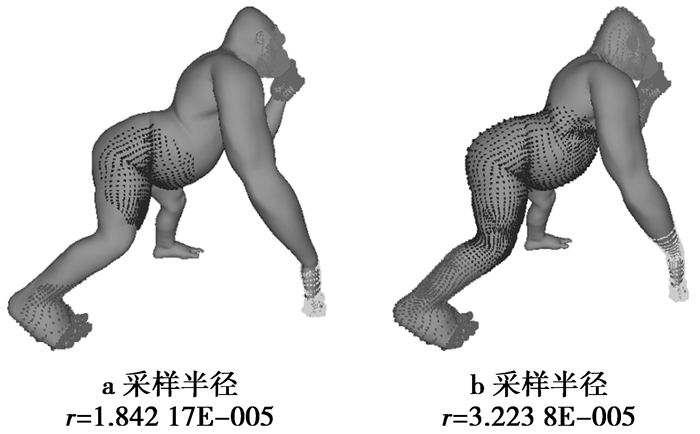图 1 不同采样半径下的COES采样结果 Figure 1 COES results under different sampling radius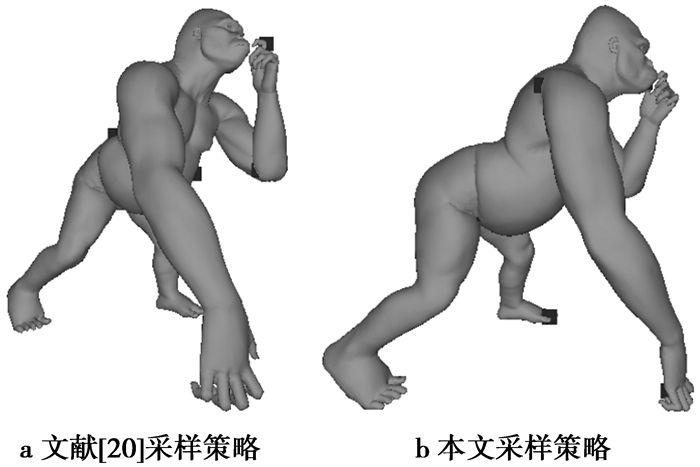图 2 不同采样策略下的COES采样结果 Figure 2 COES results under different sampling strategies
4 初始匹配

 ${U_{ij}} = \exp \left( { - {g^2}\left( {i,j} \right)/2{\sigma ^2}} \right)$ (12)

(12) 式中，σ表示模型表面上最大的测地距离长度。

 ${C_m} = \sum\limits_{i - 1}^{\left| {{s_m}} \right|} {\left( {{{\left\| {{s_{i,m}} - {t_i}} \right\|}_2}} \right)}$ (13)

(13) 式中：ti表示在植入空间中目标模型上的采样点；|sm|表示在植入空间中源模型上采样点的个数，其中，m表示在植入空间中源模型上每个采样点的邻接点个数。

 $d_{{\rm{iso}}}^{\left( 0 \right)}\left( {{s_i},{t_j}} \right) = {\left\| {{{s'}_i} - {{t'}_j}} \right\|_2}$ (14)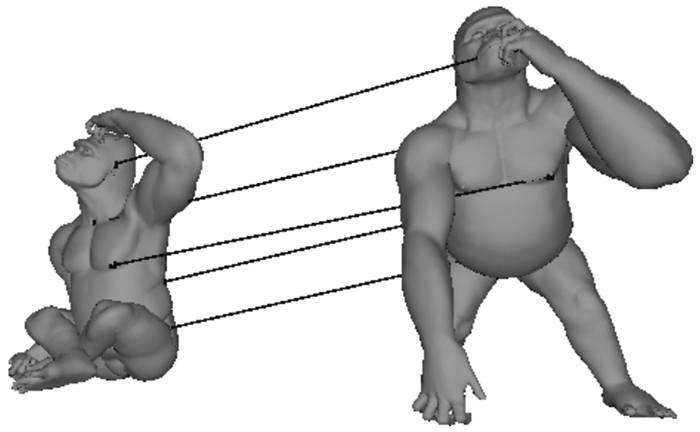图 3 猩猩模型初始匹配阶段的稀疏对应关系 Figure 3 Initial sparse correspondence between gorilla pairs
5 稠密对应关系计算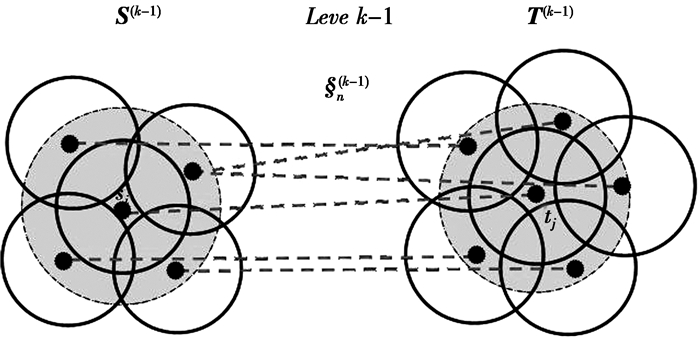图 4 计算第k-1层对应关系 Figure 4 Calculation of the k-1th level shape correspondences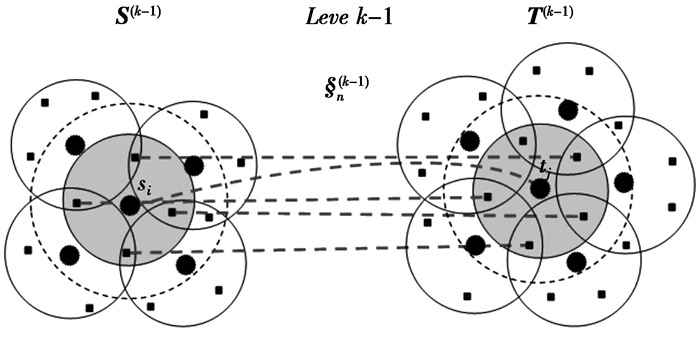图 5 计算第k层对应关系 Figure 5 Calculation of the kth level shape correspondences
6 实验结果与分析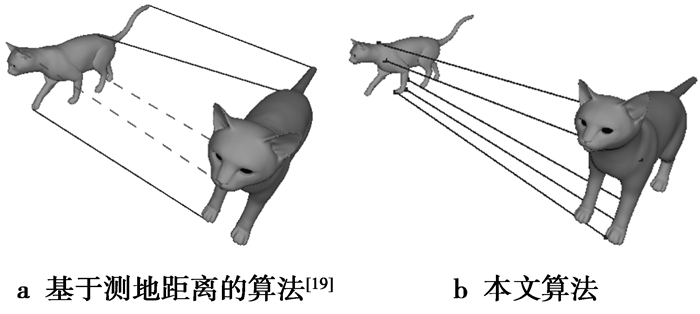图 6 构建猫模型的初始对应关系比较 Figure 6 Comparison of initial correspondences between cat pairs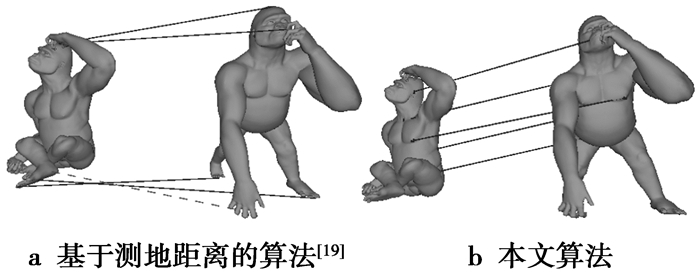图 7 构建猩猩模型的初始对应关系比较 Figure 7 Comparison of initial correspondences between gorilla pairs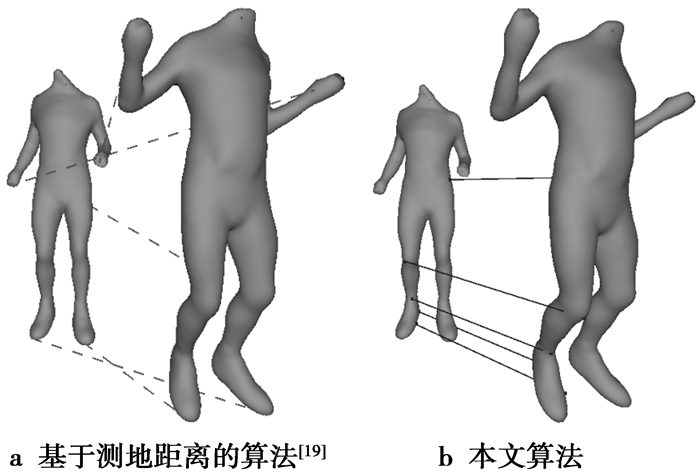图 8 构建人体模型的初始对应关系比较 Figure 8 Comparison of initial correspondences between human pairs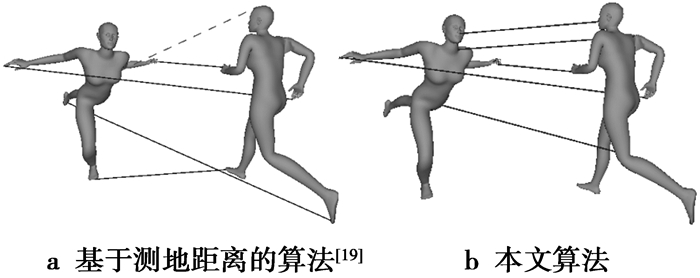图 9 构建跳芭蕾的女演员模型的初始对应关系比较 Figure 9 Comparison of initial correspondences between ballerina pairs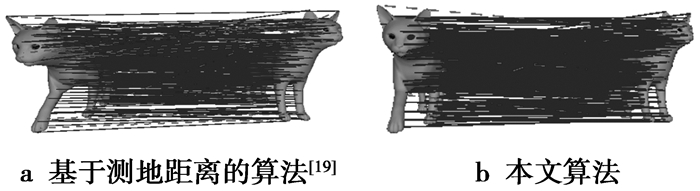图 10 计算猫模型的稠密对应关系比较 Figure 10 Comparison of dense correspondences between cat pairs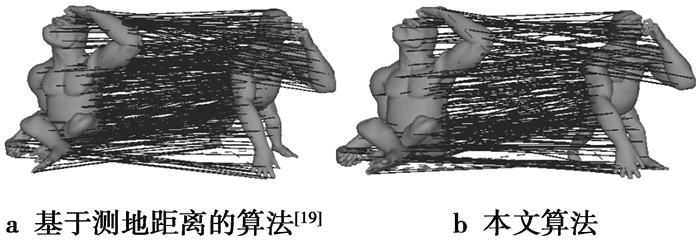图 11 计算猩猩模型的稠密对应关系比较 Figure 11 Comparison of dense correspondences between gorilla pairs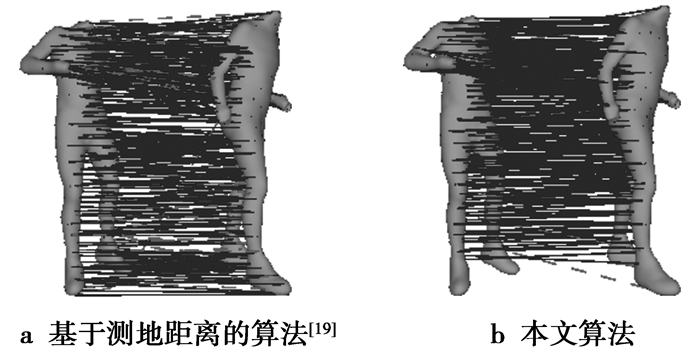图 12 计算人体模型的稠密对应关系比较 Figure 12 Comparison of dense correspondences between human pairs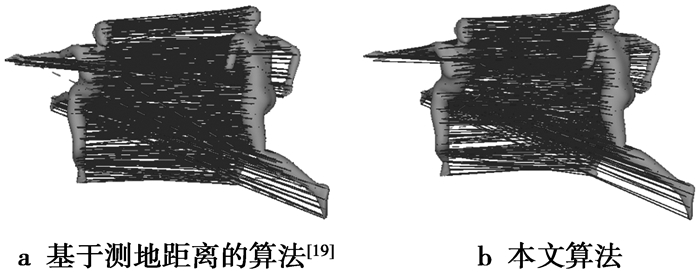图 13 计算跳芭蕾的女演员模型的对应关系比较 Figure 13 Comparison of dense correspondences between ballerina pairs表 1 2种方法计算的模型间等距误差的比较 Table 1 Comparison of the isometric error of the two kinds of methods for calculating shape correspondences between models
7 结束语

  van KAICK O, ZHANG H, HAMARNEH G, et al. A survey on shape correspondence[J]. Computer Graphics Forum, 2011, 30(6): 1681-1707. DOI:10.1111/cgf.2011.30.issue-6  KILIAN M, MITRAN N J, POTTMANN H. Geometric modeling in shape space[J]. ACM Transactions on Graphics, 2007, 26(3): 64-72. DOI:10.1145/1276377  BERKITEN S, HALBER M, SOLOMON J, et al. Learning detail transfer based on geometric features[J]. Computer Graphics Forum, 2017, 36(2): 361-373. DOI:10.1111/cgf.2017.36.issue-2  CHEN Q, KOLTUN V. Robust nonrigid registration by convex optimization[C]//IEEE International Conference on Computer Vision Workshop.Santiago, Chile: IEEE Press, 2015: 2039-2047.  REN K Y, SUN H X, JIA Q X, et al. Urban scene recognition by graphical model and 3D geometry[J]. The Journal of China Universities of Posts and Telecommunications, 2011, 18(3): 110-119. DOI:10.1016/S1005-8885(10)60072-6  NOGNENG D, OVSJANIKOV M. Informative descriptor preservation via commutativity for shape matching[J]. Computer Graphics Forum, 2017, 36(2): 259-267. DOI:10.1111/cgf.2017.36.issue-2  RAVIV D, BRONSTEIN A M, BRONSTEIN M M, et al. Equi-affine invariant geometry for shape analysis[J]. Journal of Mathematical Imaging & Vision, 2014, 50(1-2): 144-163.  MASCI J, BOSCAINI D, BRONSTEIN M M, et al. Geodesic convolutional neural networks on riemannian manifolds[C]//IEEE International Conference on Computer Vision Workshop.Santiago, Chile: IEEE Press, 2015: 832-840.  YEMEZ Y. Multiple shape correspondence by dynamic programming[J]. Computer Graphics Forum, 2015, 33(7): 121-130.  LIPMAN Y, FUNKHOUSER T A. Mobius voting for surface correspondence[J]. ACM Transactions on Graphics, 2009, 28(3): 1-12.  ZENG Y, WANG C, WANG Y, et al. Dense non-rigid surface registration using high-order graph matching[J]. Computer Vision and Pattern Recognition, 2010, 23(3): 382-389.  KIM V G, LIPMAN Y, FUNKHOUSER T. Blended intrinsic maps[J]. ACM Transactions on Graphics, 2011, 30(4): 1-12.  AFLALO Y, DUBROVINA A, KIMMEL R. Spectral generalized multi-dimensional scaling[J]. International Journal of Computer Vision, 2013, 118(3): 1-13.  杨军, 李龙杰, 田振华, 等. 非刚性变换的三维等距模型的对应关系研究[J]. 计算机科学与探索, 2014, 8(8): 1009-1016. YANG Jun, LI Longjie, TIAN Zhenhua, et al. Research on shape correspondence of 3D isometric models differing by non-rigid deformations[J]. Journal of Frontiers of Computer Science and Technology, 2014, 8(8): 1009-1016.  杨军, 闫寒, 王茂正. 融合特征描述符约束的3维等距模型对应关系计算[J]. 中国图象图形学报, 2016, 21(5): 628-635. YANG Jun, YAN Han, WANG Maozheng. Calculation of correspondences between three-dimensional isometric shapes with the use of a fused feature descriptor[J]. Journal of Image and Graphics, 2016, 21(5): 628-635.  GANAPATHI-SUBRAMANIAN V, THIBERT B, OVSJANIKOV M, et al. Stable region correspondences between non-isometric shapes[J]. Computer Graphics Forum, 2016, 35(5): 121-133. DOI:10.1111/cgf.2016.35.issue-5  HUANG H, KALOGERAKIS E, CHAUDHURI S, et al. Learning local shape descriptors from part correspondences with multi-view convolutional networks[J]. ACM Transactions on Graphics, 2017, 37(1): 1-14.  SAHILLIOĞLU Y, YEMEZ Y. Minimum distortion isometric shape correspondence using EM algorithm[J]. IEEE Transactions on Pattern Analysis & Machine Intelligence, 2012, 34(11): 2203-2215.  SAHILLIOĞLU Y, YEMEZ Y. Coarse-to-fine combinatorial matching for dense isometric shape correspondence[J]. Computer Graphics Forum, 2011, 30(5): 1461-1470. DOI:10.1111/cgf.2011.30.issue-5  HILAGA M, KOHMURA T, KOHMURA T, et al. Topology matching for fully automatic similarity estimation of 3D shapes[C]// Proceeding of the 28th Annual Conference on Computer Graphics and Interactive Techniques. New York, NY, USA: ACM Press, 2001: 203-212.